# Collecting Like Terms Worksheet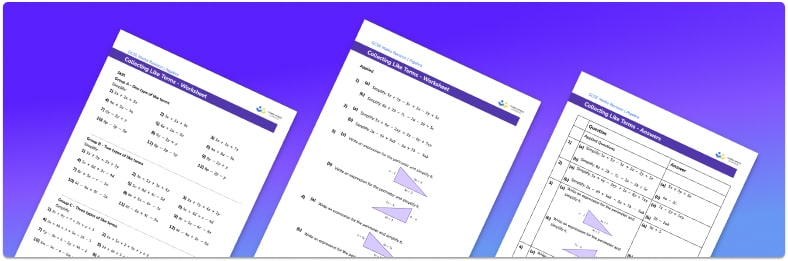• This field is for validation purposes and should be left unchanged.

You can unsubscribe at any time (each email we send will contain an easy way to unsubscribe). To find out more about how we use your data, see our privacy policy.

• Section 1 of the collecting like terms worksheet contains 48 skills-based factorising questions, in 4 groups to support differentiation
• Section 2 contains 4 applied collecting like terms questions with a mix of worded problems and deeper problem solving questions
• Section 3 contains 3 foundation and higher level GCSE exam style collecting like terms questions
• Answers and a mark scheme for all collecting like terms questions are provided
• Questions follow variation theory with plenty of opportunities for students to work independently at their own level
• All questions created by fully qualified expert secondary maths teachers
• Suitable for GCSE maths revision for AQA, OCR and Edexcel exam boards

### Collecting like terms at a glance

Collecting like terms combines algebraic terms and constant terms to simplify an expression. We use addition and subtraction of the coefficients to simplify the algebraic expression to a single term. The coefficients are usually integers, but may be decimals or fractions. Understanding the order of operations is useful when collecting like terms.

Collecting like terms moves onto more complex expressions including constants and terms involving powers (or indices). One way to do this is to rearrange the expression to group the like terms together. This will sometimes result in an algebraic expression with two terms, which is called a binomial.

Collecting like terms can be applied to other situations such as geometry. An example of this would be being given the sides of polygons and being asked to write a simplified expression for the perimeter.

Collecting like terms is a valuable skill which is needed to support work on other topics such as linear equations, quadratic equations or rearranging formulae.

Looking forward, students can then progress to additional algebra worksheets, for example a simultaneous equations worksheet or a solving equations worksheet.For more teaching and learning support on Algebra our GCSE maths lessons provide step by step support for all GCSE maths concepts step by step.

Topics covered include algebraic expressions, linear equations, quadratic equations and algebraic fractions. Suitable for use by teachers and students as preparation for GCSE before A level.

## Related worksheets

Algebraic Fractions Worksheet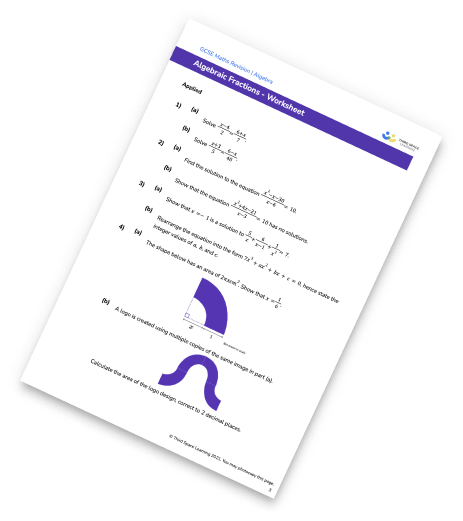Expand and Simplify Worksheet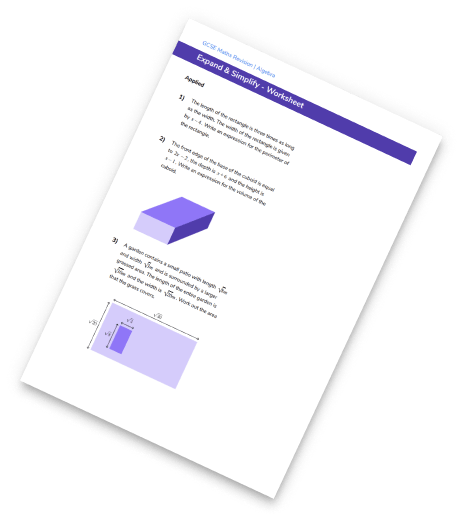Algebraic Expressions Worksheet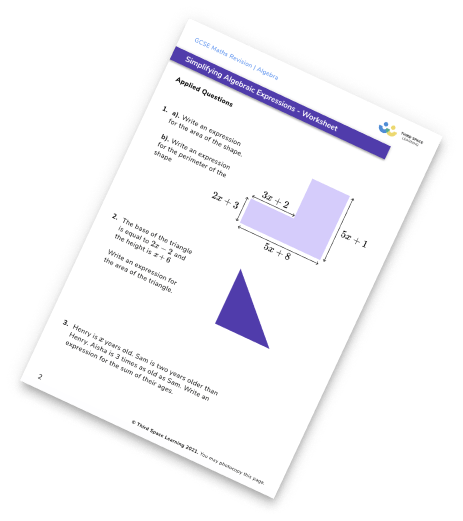Simplifying Expressions Worksheet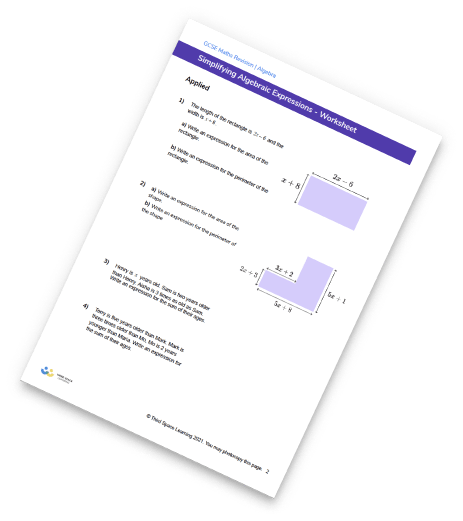## Do you have KS4 students who need more focused attention to succeed at GCSE?There will be students in your class who require individual attention to help them succeed in their maths GCSEs. In a class of 30, it’s not always easy to provide.

Help your students feel confident with exam-style questions and the strategies they’ll need to answer them correctly with our dedicated GCSE maths revision programme.

Lessons are selected to provide support where each student needs it most, and specially-trained GCSE maths tutors adapt the pitch and pace of each lesson. This ensures a personalised revision programme that raises grades and boosts confidence.

Find out more